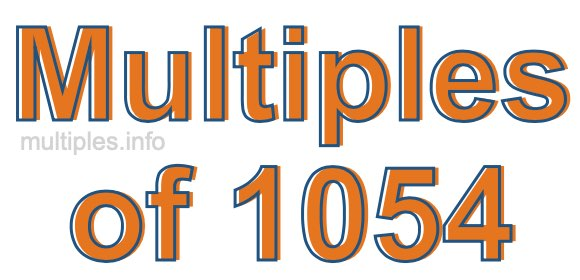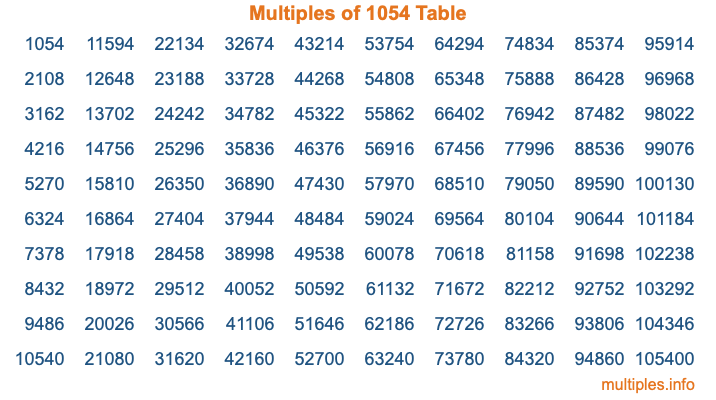Multiples of 1054Welcome to the Multiples of 1054 page. Here we will first teach you everything you will ever need to know about the multiples of 1054, and then give you a study guide summary of everything we taught you to make sure you remember it all. Use this page to look up facts and learn information about the multiples of 1054. This page will make you a multiples of one thousand fifty-four expert!

Definition of Multiples of 1054
Multiples of 1054 are all the numbers that when divided by 1054 equal an integer. Each of the multiples of 1054 are called a multiple. A multiple of 1054 is created by multiplying 1054 by an integer.

Therefore, to create a list of multiples of 1054, you start with 1 multiplied by 1054, then 2 multiplied by 1054, then 3 multiplied by 1054, and so on for as long as you want. Thus, the list of the first five multiples of 1054 is 1054, 2108, 3162, 4216, and 5270. To see a larger list of multiples of 1054, see the printable image of Multiples of 1054 further down on this page. We also have a category where you can choose any nth multiple of 1054.

Multiples of 1054 Checker
The Multiples of 1054 Checker below checks to see if any number of your choice is a multiple of 1054. In other words, it checks to see if there is any number (integer) that when multiplied by 1054 will equal your number. To do that, we divide your number by 1054. If the the quotient is an integer, then your number is a multiple of 1054.

Is  a multiple of 1054?

Least Common Multiple of 1054 and ...
A Least Common Multiple (LCM) is the lowest multiple that two or more numbers have in common. This is also called the smallest common multiple or lowest common multiple and is useful to know when you are adding our subtracting fractions. Enter one or more numbers below (1054 is already entered) to find the LCM.

Check out our LCM Calculator if you need more details about the Least Common Multiple or if you need the LCM for different numbers for adding and subtraction fractions.

nth Multiple of 1054
As we stated above, 1054 is the first multiple of 1054, 2108 is the second multiple of 1054, 3162 is the third multiple of 1054, and so on. Enter a number below to find the nth multiple of 1054.

th multiple of 1054

Multiples of 1054 vs Factors of 1054
1054 is a multiple of 1054 and a factor of 1054, but that is where the similarities end. All postive multiples of 1054 are 1054 or greater than 1054. All positive factors of 1054 are 1054 or less than 1054.

Below is the beginning list of multiples of 1054 and the factors of 1054 so you can compare:

Multiples of 1054: 1054, 2108, 3162, 4216, 5270, etc.

Factors of 1054: 1, 2, 17, 31, 34, 62, 527, 1054

As you can see, the multiples of 1054 are all the numbers that you can divide by 1054 to get a whole number. The factors of 1054, on the other hand, are all the whole numbers that you can multiply by another whole number to get 1054.

It's also interesting to note that if a number (x) is a factor of 1054, then 1054 will also be a multiple of that number (x).

Multiples of 1054 vs Divisors of 1054
The divisors of 1054 are all the integers that 1054 can be divided by evenly. Below is a list of the divisors of 1054.

Divisors of 1054: 1, 2, 17, 31, 34, 62, 527, 1054

The interesting thing to note here is that if you take any multiple of 1054 and divide it by a divisor of 1054, you will see that the quotient is an integer.

Multiples of 1054 Table
Below is an image of the first 100 multiples of 1054 in a table. The table is in chronological order, column by column. The first column has the first ten multiples of 1054, the second column has the next ten multiples of 1054, and so on.The Multiples of 1054 Table is also referred to as the 1054 Times Table or Times Table of 1054. You are welcome to print out our table for your studies.

Negative Multiples of 1054
Although not often discussed or needed in math, it is worth mentioning that you can make a list of negative multiples of 1054 by multiplying 1054 by -1, then by -2, then by -3, and so on, to get the following list of negative multiples of 1054:

-1054, -2108, -3162, -4216, -5270, etc.

Multiples of 1054 Summary
Below is a summary of important Multiples of 1054 facts that we have discussed on this page. To retain the knowledge on this page, we recommend that you read through the summary and explain to yourself or a study partner why they hold true.

There are an infinite number of multiples of 1054.

A multiple of 1054 divided by 1054 will equal a whole number.

1054 divided by a factor of 1054 equals a divisor of 1054.

The nth multiple of 1054 is n times 1054.

The largest factor of 1054 is equal to the first positive multiple of 1054.

1054 is a multiple of every factor of 1054.

1054 is a multiple of 1054.

A multiple of 1054 divided by a divisor of 1054 equals an integer.

1054 divided by a divisor of 1054 equals a factor of 1054.

Any integer times 1054 will equal a multiple of 1054.

Multiples of a Number
Here you can get the multiples of another number, all with the same attention to detail as we did for multiples of 1054 on this page.

Multiples of
Multiples of 1055
Did you find our page about multiples of one thousand fifty-four educational? Do you want more knowledge? Check out the multiples of the next number on our list!